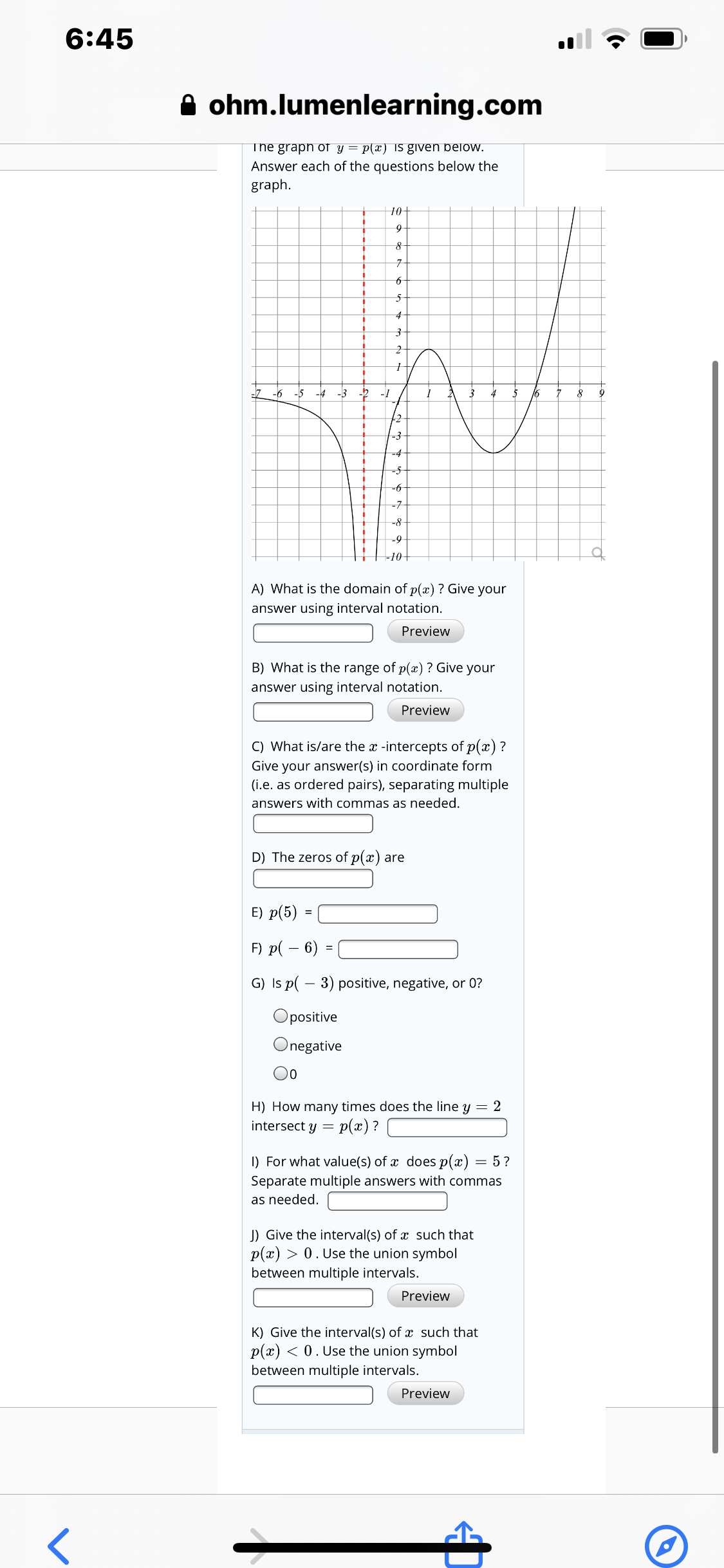# 6:45 A ohm.lumenlearning.com The graph of y = p(x) IS given below. Answer each of the questions below the graph. 10 5- -5 -4 -3 -1 16 +2 -3 -4 -6 -7- -8 -9 -10+ A) What is the domain of p(x) ? Give your answer using interval notation. Preview B) What is the range of p(æ) ? Give your answer using interval notation. Preview C) What is/are the x -intercepts of p(x) ? Give your answer(s) in coordinate form (i.e. as ordered pairs), separating multiple answers with commas as needed. D) The zeros of p(x) are E) p(5) = F) p( – 6) = G) Is p( – 3) positive, negative, or 0? Opositive Onegative 00 H) How many times does the line y = 2 p(x) ? intersect y = I) For what value(s) of x does p(x) = 5? Separate multiple answers with commas as needed. J) Give the interval(s) of x such that p(x) > 0. Use the union symbol between multiple intervals. Preview K) Give the interval(s) of x such that p(x) < 0. Use the union symbol between multiple intervals. Preview

Question

Hey can I get the bottom part answers D-khelp_outlineImage Transcriptionclose6:45 A ohm.lumenlearning.com The graph of y = p(x) IS given below. Answer each of the questions below the graph. 10 5- -5 -4 -3 -1 16 +2 -3 -4 -6 -7- -8 -9 -10+ A) What is the domain of p(x) ? Give your answer using interval notation. Preview B) What is the range of p(æ) ? Give your answer using interval notation. Preview C) What is/are the x -intercepts of p(x) ? Give your answer(s) in coordinate form (i.e. as ordered pairs), separating multiple answers with commas as needed. D) The zeros of p(x) are E) p(5) = F) p( – 6) = G) Is p( – 3) positive, negative, or 0? Opositive Onegative 00 H) How many times does the line y = 2 p(x) ? intersect y = I) For what value(s) of x does p(x) = 5? Separate multiple answers with commas as needed. J) Give the interval(s) of x such that p(x) > 0. Use the union symbol between multiple intervals. Preview K) Give the interval(s) of x such that p(x) < 0. Use the union symbol between multiple intervals. Preview fullscreen

### Want to see this answer and more?

Experts are waiting 24/7 to provide step-by-step solutions in as fast as 30 minutes!*

*Response times may vary by subject and question complexity. Median response time is 34 minutes for paid subscribers and may be longer for promotional offers.
Tagged in
Math
Algebra

### Other